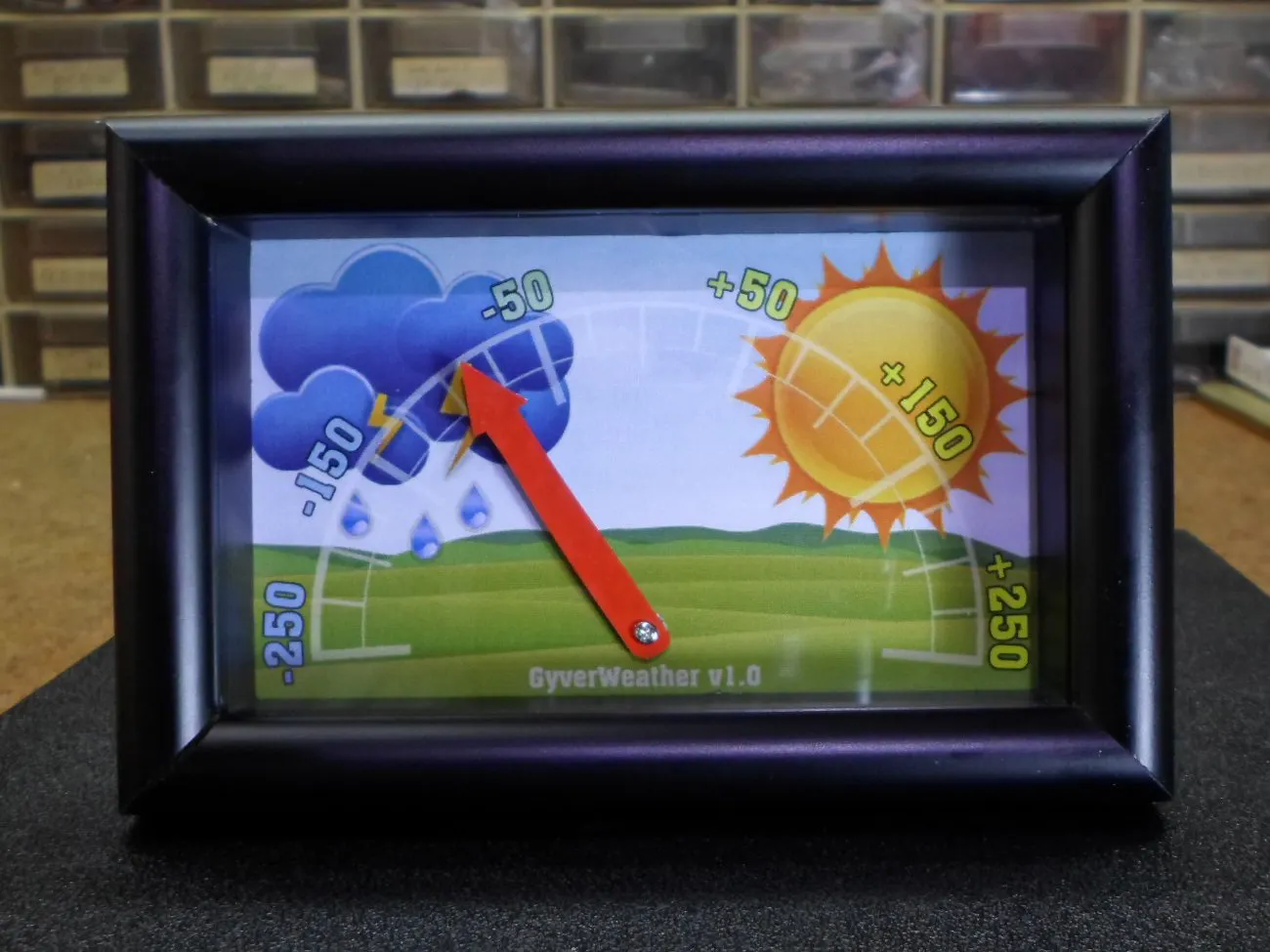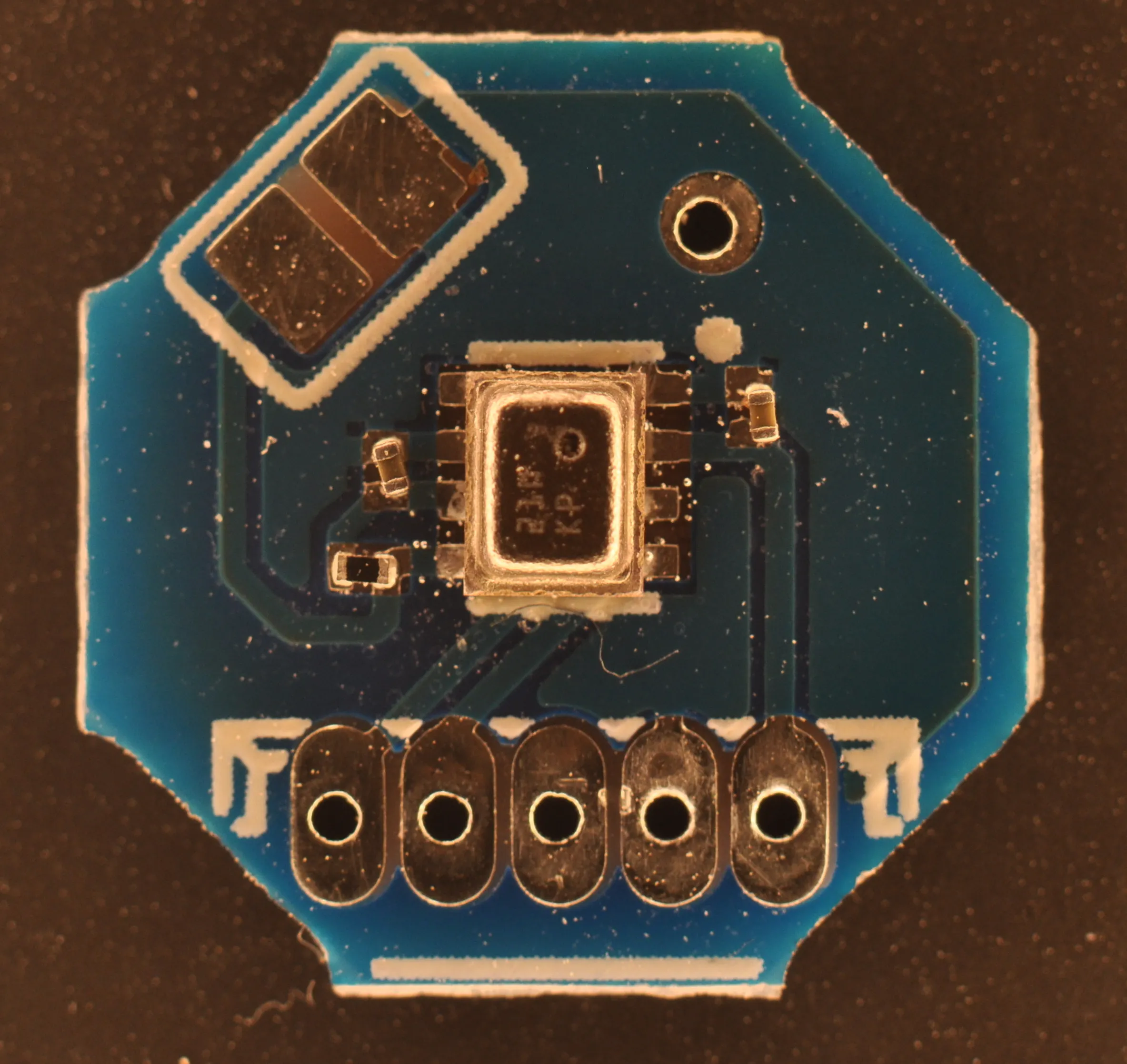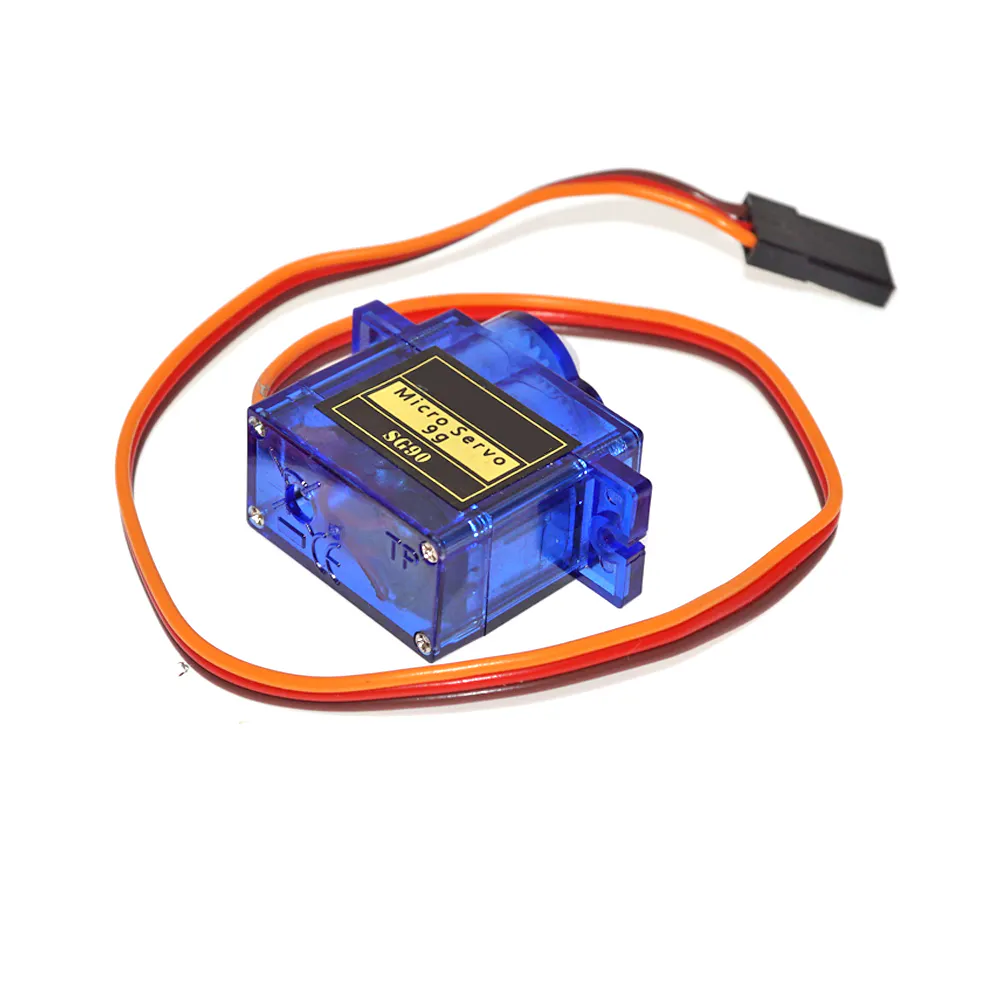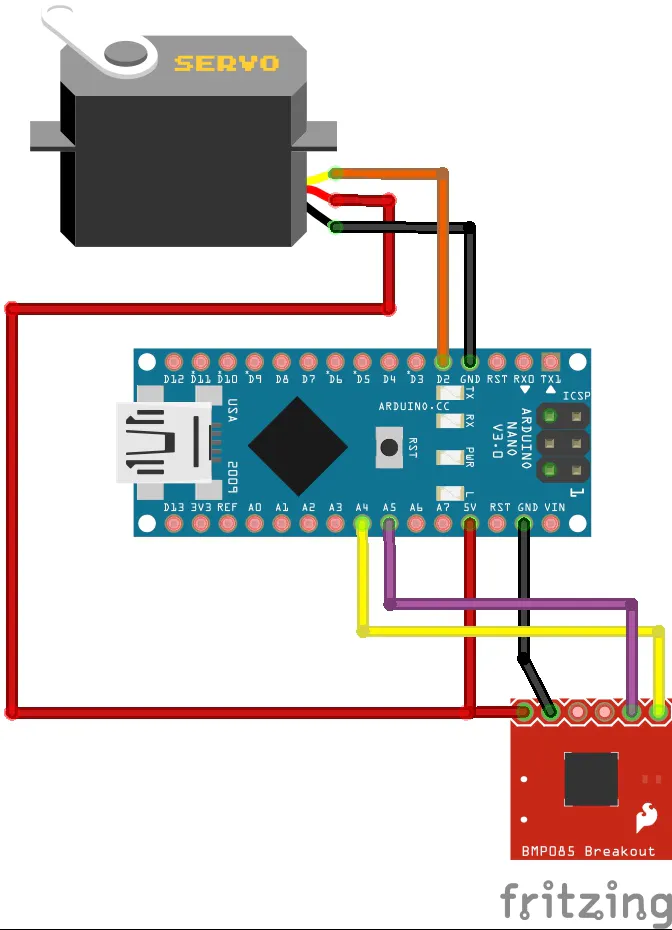# DIY Simple Weather Forecast Device

Great instrument for short time local weather forecasting. Uses only Arduino, BMP180 and 9g servo.

BeginnerFull instructions provided5,486## Things used in this project

### Hardware componentsArduino Nano R3
×1MyOctopus i2c Barometric Air Pressure Sensor BMP280
×1SG90 Micro-servo motor
×1

### Software apps and online servicesArduino IDE

### Hand tools and fabrication machinesSoldering iron (generic)

## Schematics

### Schematic diagram## Code

### Arduino code

C/C++
```/*
Электронный предсказатель погоды по изменению давления
Измеряет давление каждые 10 минут, находит разницу с давлением час назад
При расчёте давления использовано среднее арифметическое из 10 измерений
для уменьшения шумности сигнала с датчика

AlexGyver 2017
*/

//-----------------------НАСТРОЙКИ---------------------
#define servo_invert 1       // если серва крутится не в ту сторону, изменить значение (1 на 0, 0 на 1)
#define battery_min 3000     // минимальный уровень заряда батареи для отображения
#define battery_max 5200     // максимальный уровень заряда батареи для отображения
// диапазон для 3 пальчиковых/мизинчиковых батареек: 3000 - 4700
// диапазон для одной банки литиевого аккумулятора: 3000 - 4200
//-----------------------НАСТРОЙКИ---------------------

#define servo_Vcc 12           // пин питания, куда подключен мосфет

//------ИНВЕРСИЯ------
#if servo_invert == 1
#define servo_180 0
#define servo_0 180
#else
#define servo_0 0
#define servo_180 180
#endif
//------ИНВЕРСИЯ------

//------БИБЛИОТЕКИ------
#include <Servo.h>             // библиотека серво
#include <Wire.h>              // вспомогательная библиотека датчика
#include <LowPower.h>          // библиотека сна
//------ИНВЕРСИЯ------

boolean wake_flag, move_arrow;
int sleep_count, angle, delta, last_angle = 90;
float k = 0.8;
float my_vcc_const = 1.080;    // константа вольтметра
unsigned long pressure, aver_pressure, pressure_array, time_array;
unsigned long sumX, sumY, sumX2, sumXY;
float a, b;

Servo servo;
Adafruit_BMP085 bmp; //объявить датчик с именем bmp

void setup() {
Serial.begin(9600);
pinMode(servo_Vcc, OUTPUT);
pinMode(A3, OUTPUT);
pinMode(A2, OUTPUT);
digitalWrite(servo_Vcc, 1);      // подать питание на серво
digitalWrite(A3, 1);             // подать питание на датчик
digitalWrite(A2, 0);
delay(500);
bmp.begin(BMP085_ULTRAHIGHRES);  // включить датчик
servo.attach(2);                 // подключить серво
servo.write(servo_0);            // увести серво в крайнее левое положение
delay(1000);
int voltage = readVcc();         // считать напряжение питания

// перевести его в диапазон поворота вала сервомашинки
voltage = map(voltage, battery_min, battery_max, servo_0, servo_180);
voltage = constrain(voltage, 0, 180);
servo.write(voltage);            // повернуть серво на угол заряда
delay(3000);
servo.write(90);                 // поставить серво в центр
delay(2000);
digitalWrite(servo_Vcc, 0);      // отключить серво
pressure = aver_sens();          // найти текущее давление по среднему арифметическому
for (byte i = 0; i < 6; i++) {   // счётчик от 0 до 5
pressure_array[i] = pressure;  // забить весь массив текущим давлением
time_array[i] = i;             // забить массив времени числами 0 - 5
}
}

void loop() {
if (wake_flag) {
delay(500);
pressure = aver_sens();                          // найти текущее давление по среднему арифметическому
for (byte i = 0; i < 5; i++) {                   // счётчик от 0 до 5 (да, до 5. Так как 4 меньше 5)
pressure_array[i] = pressure_array[i + 1];     // сдвинуть массив давлений КРОМЕ ПОСЛЕДНЕЙ ЯЧЕЙКИ на шаг назад
}
pressure_array = pressure;                    // последний элемент массива теперь - новое давление

sumX = 0;
sumY = 0;
sumX2 = 0;
sumXY = 0;
for (int i = 0; i < 6; i++) {                    // для всех элементов массива
sumX += time_array[i];
sumY += (long)pressure_array[i];
sumX2 += time_array[i] * time_array[i];
sumXY += (long)time_array[i] * pressure_array[i];
}
a = 0;
a = (long)6 * sumXY;             // расчёт коэффициента наклона приямой
a = a - (long)sumX * sumY;
a = (float)a / (6 * sumX2 - sumX * sumX);
// Вопрос: зачем столько раз пересчитывать всё отдельными формулами? Почему нельзя считать одной большой?
// Ответ: а затем, что ардуинка не хочет считать такие большие числа сразу, и обязательно где-то наё*бывается,
// выдавая огромное число, от которого всё идёт по пи*зде. Почему с матами? потому что устал отлаживать >:O
delta = a * 6;                   // расчёт изменения давления

angle = map(delta, -250, 250, servo_0, servo_180);  // пересчитать в угол поворота сервы
angle = constrain(angle, 0, 180);                   // ограничить диапазон

// дальше такая фишка: если угол несильно изменился с прошлого раза, то нет смысла лишний раз включать серву
// и тратить энергию/жужжать. Так что находим разницу, и если изменение существенное - то поворачиваем стрелку
if (abs(angle - last_angle) > 7) move_arrow = 1;

if (move_arrow) {
last_angle = angle;
digitalWrite(servo_Vcc, 1);      // подать питание на серво
delay(300);                      // задержка для стабильности
servo.write(angle);              // повернуть серво
delay(1000);                     // даём время на поворот
digitalWrite(servo_Vcc, 0);      // отключить серво
move_arrow = 0;
}

if (readVcc() < battery_min) LowPower.powerDown(SLEEP_FOREVER, ADC_OFF, BOD_OFF); // вечный сон если акум сел
wake_flag = 0;
delay(10);                       // задержка для стабильности
}

LowPower.powerDown(SLEEP_8S, ADC_OFF, BOD_OFF);      // спать 8 сек. mode POWER_OFF, АЦП выкл
sleep_count++;            // +1 к счетчику просыпаний
if (sleep_count >= 70) {  // если время сна превысило 10 минут (75 раз по 8 секунд - подгон = 70)
wake_flag = 1;          // рарешить выполнение расчета
sleep_count = 0;        // обнулить счетчик
delay(2);               // задержка для стабильности
}
}

// среднее арифметичсекое от давления
long aver_sens() {
pressure = 0;
for (byte i = 0; i < 10; i++) {
}
aver_pressure = pressure / 10;
return aver_pressure;
}

long readVcc() { //функция чтения внутреннего опорного напряжения, универсальная (для всех ардуин)
#if defined(__AVR_ATmega32U4__) || defined(__AVR_ATmega1280__) || defined(__AVR_ATmega2560__)
ADMUX = _BV(REFS0) | _BV(MUX4) | _BV(MUX3) | _BV(MUX2) | _BV(MUX1);
#elif defined (__AVR_ATtiny24__) || defined(__AVR_ATtiny44__) || defined(__AVR_ATtiny84__)
#elif defined (__AVR_ATtiny25__) || defined(__AVR_ATtiny45__) || defined(__AVR_ATtiny85__)
#else
ADMUX = _BV(REFS0) | _BV(MUX3) | _BV(MUX2) | _BV(MUX1);
#endif
delay(2); // Wait for Vref to settle
uint8_t high = ADCH; // unlocks both
long result = (high << 8) | low;

result = my_vcc_const * 1023 * 1000 / result; // расчёт реального VCC
return result; // возвращает VCC
}
```

## Credits

### Mirko Pavleski

18 projects • 476 followers